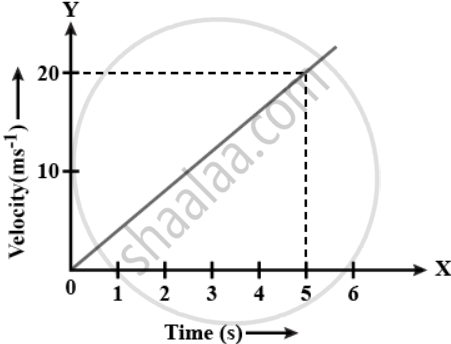# Figure shows the velocity-time graph of a particle of mass 100 g moving in a straight line. Calculate the force acting on the particle. (Hint : Acceleration = Slope of the v-t graph) - Physics

Sum

Figure shows the velocity-time graph of a particle of mass 100 g moving in a straight line. Calculate the force acting on the particle.
(Hint : Acceleration = Slope of the v-t graph)#### Solution

Given mass, m = 100 g = 0.1 kg.

Slope of a velocity-time graph gives the value of acceleration.

Acceleration = Slope = 20/5 = 4 m/s2.

Force = Mass × Acceleration.

Force = 0.1 × 4 = 0.4 N.

Concept: Free Fall
Is there an error in this question or solution?

#### APPEARS IN

Selina Concise Physics Class 9 ICSE
Chapter 3 Laws of Motion
Exercise 3 (C) | Q 8 | Page 70